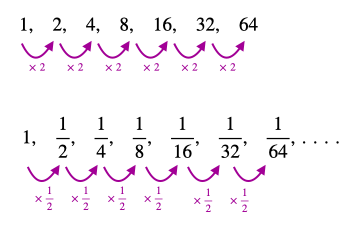407 493 6601

# Algebra 2 - Geometric Sequence & Series

## Introduction

• A geometric sequence (aka geometric progression) is a special type of sequence in which the ratio between two consecutive terms is always the same (constant).
• The ratio is called common ratio $\mathbit{\left(}\mathbit{r}\mathbit{\right)}$.
• Some examples of geometric sequences are given in the figure below.• Based on the number of terms, geometric sequences are classified into two types - finite and infinite.
• The first sequence shown in the figure above represents a finite geometric sequence (with $\mathbit{r}\mathbf{=}\mathbf{2}$) as it has a finite number of terms.
• On the other hand, the second sequence (with $\mathbit{r}\mathbf{=}\frac{\mathbf{1}}{\mathbf{2}}$) has an infinite number of terms (indicated by dots) and hence is an example of an infinite geometric sequence.
• In general, a geometric sequence is represented as: , where ${\mathbit{a}}_{\mathbf{1}}\mathbf{=}$first term and $\mathbit{r}\mathbf{=}$common ratio.
• The ${\mathbit{n}}^{\mathbf{t}\mathbf{h}}$ term of a geometric sequence is, ${\mathbit{a}}_{\mathbf{n}}\mathbf{=}{\mathbit{a}}_{\mathbf{1}}{\mathbit{r}}^{\mathbf{n}\mathbf{-}\mathbf{1}}$.

• It is the sum of the terms of a geometric sequence.
• The formula to find the sum of '$\mathbit{n}$' terms of a finite geometric sequence is ${\mathbit{S}}_{\mathbf{n}}\mathbf{=}\frac{{\mathbf{a}}_{\mathbf{1}}\mathbf{·}\mathbf{\left(}\mathbf{1}\mathbf{-}{\mathbf{r}}^{\mathbf{n}}\mathbf{\right)}}{\left(1-r\right)}$, provided $r\ne 1$.
• The formula to find the sum of '$\mathbit{n}$' terms of an infinite geometric sequence with $\mathbf{|}\mathbf{r}\mathbf{|}\mathbf{<}\mathbf{1}$ is ${\mathbit{S}}_{\mathbf{\infty }}\mathbf{=}\frac{{\mathbf{a}}_{\mathbf{1}}}{\left(1-r\right)}$.

## Solved Examples

Example 1: Is  a geometric sequence?

Solution: The condition for a sequence to be a geometric sequence is that it must have a common ratio. Since this condition is not satisfied for the given sequence, it is not a geometric sequence.

[Note: In the given sequence, the difference between any two consecutive terms is the same (common difference $\mathbit{d}\mathbf{=}\mathbf{5}$) and hence is an arithmetic sequence.]

Example 2: Find the first six terms of a geometric sequence in which the first term is ${\mathbit{a}}_{\mathbf{1}}\mathbf{=}\mathbf{3}$ and the common ratio is $\mathbit{r}\mathbf{=}\mathbf{2}$.

Solution: It is given that the first term is ${\mathbit{a}}_{\mathbf{1}}\mathbf{=}\mathbf{3}$ and the common ratio is $\mathbit{r}\mathbf{=}\mathbf{2}$. We know that the ${\mathbit{n}}^{\mathbf{t}\mathbf{h}}$ of a a geometric sequence is ${\mathbit{a}}_{\mathbf{n}}\mathbf{=}{\mathbit{a}}_{\mathbf{1}}{\mathbit{r}}^{\mathbf{n}\mathbf{-}\mathbf{1}}$. We can find the other terms using this formula.

Second term, ${a}_{2}=\left(3\right)·{\left(2\right)}^{2-1}$$=\left(3\right)·{\left(2\right)}^{1}=6$

Third term, ${a}_{3}=\left(3\right)·{\left(2\right)}^{3-1}$$=\left(3\right)·{\left(2\right)}^{2}=12$

Fourth term, ${a}_{4}=\left(3\right)·{\left(2\right)}^{4-1}$$=\left(3\right)·{\left(2\right)}^{3}=24$

Fifth term, ${a}_{5}=\left(3\right)·{\left(2\right)}^{5-1}$$=\left(3\right)·{\left(2\right)}^{4}=48$

Sixth term, ${a}_{6}=\left(3\right)·{\left(2\right)}^{6-1}$$=\left(3\right)·{\left(2\right)}^{5}=96$

Therefore, the first six terms of the given geometric sequence are $\mathbf{48}\mathbf{,}$ and.

Example 3: Find the next term of a geometric sequence

Solution: Here, the first four terms of the geometric sequence are given. The first term is, ${\mathbit{a}}_{\mathbf{1}}\mathbf{=}\mathbf{5}$. We can calculate the common ratio, $\mathbit{r}\mathbf{=}\frac{\mathbf{1}}{\mathbf{5}}$.

Now, the next term means the fifth term i.e. ${a}_{5}=\left(5\right)·{\left(\frac{1}{5}\right)}^{5-1}$$=\left(5\right)·{\left(\frac{1}{5}\right)}^{4}$$=\left(5\right)·\left(\frac{1}{5×5×5×5}\right)$$=\frac{1}{125}$

Example 4: Find the sum of the first ten terms of the given geometric sequence:

Solution: Here, the first term ${\mathbit{a}}_{\mathbf{1}}\mathbf{=}\mathbf{2}$, common ratio $\mathbit{r}\mathbf{=}\frac{\mathbf{-}\mathbf{6}}{\mathbf{2}}\mathbf{=}\mathbf{-}\mathbf{3}$. We know that, ${S}_{n}=\frac{{a}_{1}·\left(1-{r}^{n}\right)}{\left(1-r\right)}$.

${S}_{10}=\frac{\left(2\right)·\left[1-{\left(-3\right)}^{10}\right]}{\left[1-\left(-3\right)\right]}$$=\frac{\left(2\right)·\left(1-59049\right)}{\left(1+3\right)}$$=\frac{\left(2\right)·\left(-59048\right)}{4}$$=-29524$

Example 5: Find the sum of the infinite geometric series

Solution: Here, the first term ${\mathbit{a}}_{\mathbf{1}}\mathbf{=}\mathbf{27}$, common ratio $\mathbit{d}\mathbf{=}\frac{\mathbf{9}}{\mathbf{27}}\mathbf{=}\frac{\mathbf{1}}{\mathbf{3}}$. We know that for $\left|r\right|<1$${\mathbit{S}}_{\mathbf{\infty }}\mathbf{=}\frac{\mathbf{a}}{\left(1-r\right)}$.

${S}_{\infty }=\frac{27}{\left(1-\frac{1}{3}\right)}$$=\frac{27}{\left(\frac{2}{3}\right)}$$=\frac{81}{2}$

## Cheat Sheet

• The common ratio of any geometric sequence is found by dividing any term of the geometric sequence by its preceding term i.e. $\mathbit{r}\mathbf{=}\frac{{\mathbf{a}}_{\mathbf{n}}}{{\mathbf{a}}_{\mathbf{n}\mathbf{-}\mathbf{1}}}$
• ${\mathbit{n}}^{\mathbf{t}\mathbf{h}}$ term of a geometric sequence from the beginning: ${\mathbit{a}}_{\mathbf{n}}\mathbf{=}{\mathbit{a}}_{\mathbf{1}}\mathbf{·}{\mathbit{r}}^{\mathbf{n}\mathbf{-}\mathbf{1}}$
• ${\mathbit{m}}^{\mathbf{t}\mathbf{h}}$ term of a finite geometric sequence (having '$\mathbit{n}$' terms) from the end: ${\mathbit{a}}_{m}\mathbf{=}{\mathbit{a}}_{\mathbf{1}}\mathbf{·}{\mathbit{r}}^{\mathbf{n}\mathbf{-}\mathbit{m}}$
• For a finite geometric sequence, the sum of the first '$\mathbit{n}$' terms: ${\mathbit{S}}_{\mathbf{n}}\mathbf{=}\frac{{\mathbf{a}}_{\mathbf{1}}\mathbf{·}\mathbf{\left(}\mathbf{1}\mathbf{-}{\mathbf{r}}^{\mathbf{n}}\mathbf{\right)}}{\left(1-r\right)}$, where $\mathbit{r}\mathbf{\ne }\mathbf{1}$.
• For $\mathbit{r}\mathbf{=}\mathbf{1}$, the sum of the first '$\mathbit{n}$' terms of the finite geometric sequence: ${\mathbit{S}}_{\mathbf{n}}\mathbf{=}\mathbit{n}{\mathbit{a}}_{\mathbf{1}}$.
• For an infinite geometric sequence where $\mathbf{|}\mathbf{r}\mathbf{|}\mathbf{<}\mathbf{1}$, the sum of first '$\mathbit{n}$' terms: ${\mathbit{S}}_{\mathbf{\infty }}\mathbf{=}\frac{{\mathbf{a}}_{\mathbf{1}}}{\left(1-r\right)}$.
• For an infinite geometric sequence where $\mathbf{|}\mathbf{r}\mathbf{|}\mathbf{\ge }\mathbf{1}$, the sum cannot be computed.
• If  represents a set (group) of three consecutive terms of any geometric sequence, then $\mathbit{y}$ is called the geometric mean of the set, and its value is $\mathbit{y}\mathbf{=}\sqrt{\mathbf{x}\mathbf{z}}$.

## Blunder Areas

• When each term of a geometric sequence is multiplied by a non-zero number, the resulting sequence is also a geometric sequence with the same common ratio.
• The new sequence obtained by taking the reciprocal of terms of a geometric sequence is also a geometric sequence.
• If all the terms of a given geometric sequence are raised to the same power, then the resulting sequence is also a geometric sequence.